# Grains of sand in a breath

I recently saw this tweet:

This immediately didn’t sound right to me, so I decided to investigate.

The volume of the average human breath (the tidal volume) is about half a litre or 0.0005 cubic metres; one half-litre of air is about twenty-two millimoles. One mole contains 6.02×1023 particles, so 22 mmol contains 1.32×1022 particles.

Sand ranges in size, from 0.05 millimetres to one or two millimetres in diameter. Assuming a diameter of 0.5 millimetres, and that sand grains are spherical, gives each grain of sand a volume of 6.54×10?11 cubic metres. Twenty-two millimoles of sand would therefore have a volume of 867 billion cubic metres.

However, this ignores the gaps between the sand particles. The famous mathematician Carl Fredrich Gauss showed that the closest spheres can pack together is with a packing fraction of 74.0%, meaning that 26% of the space is wasted. Our sand would therefore occupy a volume of 1.17 trillion cubic metres rather than the value calculated earlier.

The surface area of the United States (all fifty states, including land and water) is 9.83 trillion square metres. This volume of sand would therefore cover the US to a depth of eleven centimetres, which is nowhere near the height of a eight-storey building. Even if we take “cover the US” to mean only the land and only the forty-eight contiguous states then we still only get to fifteen or sixteen centimetres; and even if we used the largest possible grains of sand we’d still be out by at least two orders of magnitude. The author of the article must be imagining sand grains the size of peas or something.

(Also, the article linked-to in the tweet refers to an average human breath containing 8×1022 particles, which is six times larger than the value I calculated; I think they may have confused inspiratory reserve volume with tidal volume.)

# Why using a number twice in your PIN might be a good idea

Modern smartphones use large glass touchscreen panels that show the presence of grease from hands and faces very easily. Should your phone be stolen it could be possible for the thief to discern the PIN required to unlock it by analysing these grease patterns.

If your pin was “1234” then the thief would only have to try all 24 permutations in order to guarantee being able to unlock the phone:

• {1,2,3,4} {1,2,4,3} {1,3,2,4} {1,3,4,2} {1,4,2,3} {1,4,3,2} {2,1,3,4} {2,1,4,3} {2,3,1,4} {2,3,4,1} {2,4,1,3} {2,4,3,1} {3,1,2,4} {3,1,4,2} {3,2,1,4} {3,2,4,1} {3,4,1,2} {3,4,2,1} {4,1,2,3} {4,1,3,2} {4,2,1,3} {4,2,3,1} {4,3,1,2} {4,3,2,1}

But if you had chosen “1233” then the thief would not know which number had been used twice, and would have more permutations to check:

• {1,2,3,3} {1,3,2,3} {1,3,3,2} {2,1,3,3} {2,3,1,3} {2,3,3,1} {3,1,2,3} {3,1,3,2} {3,2,1,3} {3,2,3,1} {3,3,1,2} {3,3,2,1}
• {1,2,2,3} {1,2,3,2} {1,3,2,2} {2,1,2,3} {2,1,3,2} {2,2,1,3} {2,2,3,1} {2,3,1,2} {2,3,2,1} {3,1,2,2} {3,2,1,2} {3,2,2,1}
• {1,1,2,3} {1,1,3,2} {1,2,1,3} {1,2,3,1} {1,3,1,2} {1,3,2,1} {2,1,1,3} {2,1,3,1} {2,3,1,1} {3,1,1,2} {3,1,2,1} {3,2,1,1}

By choosing a PIN with a repeating digit you have made it 50% harder for the thief. It is also possible that the thief might not realise that a digit had been repeated, and then have to guess at the fourth digit, which would make life much harder. If this idea is extended to a 5-digit PIN then the increase in difficulty becomes 100% – it is twice as difficult with a repeated digit as without one. For every digit added it becomes fifty percentage points more difficult.

# Understanding Euler’s Identity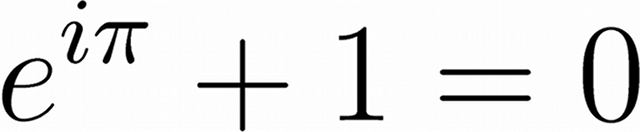Euler’s Identity, shown above, is often said to be the most beautiful equation in all of science and mathematics.

It links the three basic arithmetic operations:

• Addition, in the +1 term.
• Multiplication, in the iπ term.
• Exponentiation, in the eiπ term.

It also links five of the most important mathematical constants:

• e, the base of the natural logarithms.
• i, the imaginary number (√−1) on which complex numbers are based.
• π, the ratio of a circle’s circumference to its diameter.
• 1, the multiplicative identity, the basic unit of counting.
• 0, the additive identity that leaves a number unchanged.

Understanding how eiπ + 1 can equal zero is more difficult.

To begin to understand how to evaluate Euler’s Identity we must first understand about series expansions. The series expansion of a function can be used to calculate the value of this function to an arbitrary degree of precision.

The series expansion of ex is given by: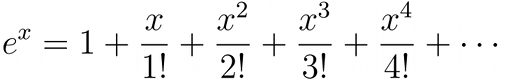If only the first term is used then e = 1.00, if two terms are used e = 2.00, if three terms are used e = 2.50, if four terms are used e = 2.66, and so on, until an infinite number of terms are used and the exact value of e is given. After only ten terms the value found for e is accurate to better than one part in a million.

The series expansion of eiπ is therefore given by: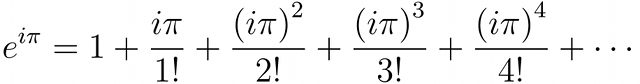The even powered terms (e.g. i2π2, i4π4) become negative because i2=−1 and the odd powered terms (e.g. i3π3, i5π5) gain a negative multiple of i.

There are two other important expansions, the expansion of sin(x) and cos(x), two basic trigonometric functions.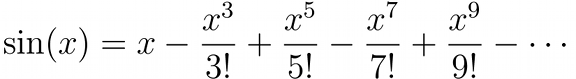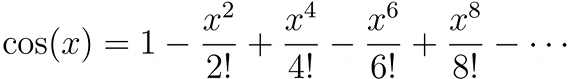If we collect the terms in the expansions of sin(x) and cos(x) and compare them with the expansion of eix we find that: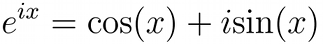Inserting π in place of x in that expression yields:Because cos(π) = −1 and sin(π) = 0 we find that indeed, e = −1 and therefore eiπ + 1 = 0.

# Making art with the Travelling Salesman Problem

The Travelling Salesman Problem is a classic problem in mathematics. The objective of the problem is to find the shortest possible route between a number of cities that visits each city only once and returns to the starting point.

Below is a rendering of the text “MrReid.org” created by solving the Travelling Salesman Problem. If you look carefully you can see that in each case the text is composed of one single line, drawn “without lifting the pen from the page”.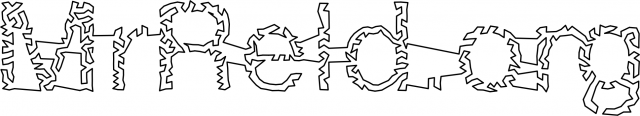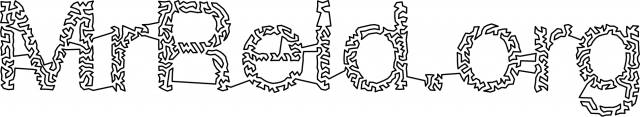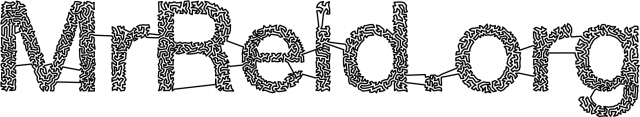Top to bottom: “MrReid.org” rendered using 1000, 2000, and 5000 nodes. Click to enlarge.

By taking a greyscale image, and turning it into a weighted Voronoi diagram (using Adrian Secord’s algorithm) it is possible to create a “map” in which the “cities” are placed closer together in dark areas and further apart in light ones.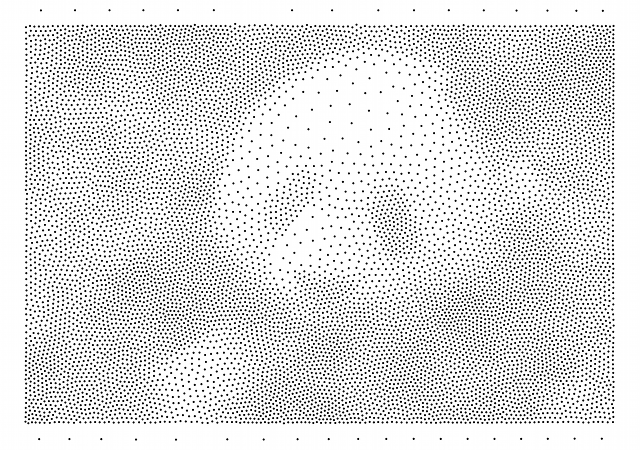Top: the original panda image. Bottom: weighted Voronoi diagram of panda image (10000 nodes).

By solving the Travelling Salesman Problem for this “map” (using the Nearest Neighbour algorithm for the first pass and and the 2-opt algorithm for subsequent optimisation) the following “route” is produced.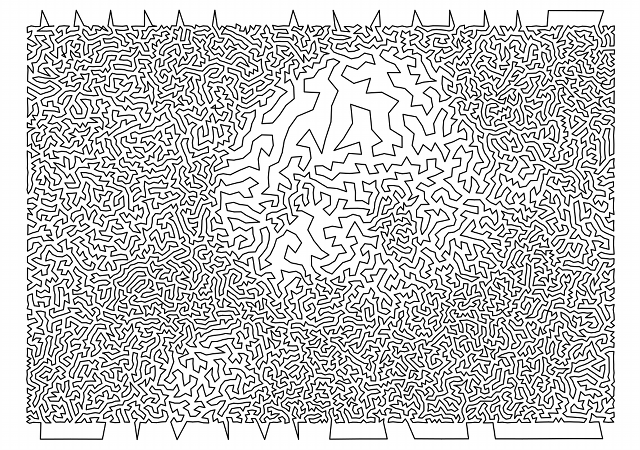There isn’t really enough contrast between the background and foreground in the original panda image, but it works if you squint a little bit.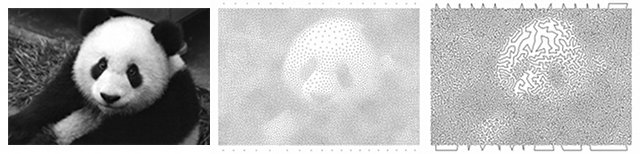# Understanding the problem with RSA

Recent reports suggest that the very commonly used RSA encryption algorithm has significant security flaws. I couldn’t find a good explanation of the mathematical problem that causes these flaws online, but I think I’ve worked it out below.

The security of RSA keys rests on the difficulty of factorising the product (usually called n) of two large primes (usually called p and q). Multiplying p and q together is a very quick operation, but working out which p and q were multipled multiplied together to make a given n is very time consuming. If you don’t believe me, see how quickly you can multiply 3259 and 6553; and compare this with the time it takes you to work out which two prime numbers were multiplied together to make 60557843.

In cryptography, n is the public key and is made widely available, whereas p and q make up the private key and must be kept secret. Public keys are usually published to keyservers (here’s mine) and this means that they can be freely downloaded.

Researchers from the Ecole Polytechnique Fédérale de Lausanne in Switzerland downloaded 11.4 million RSA keys and discovered that a number of RSA public keys share a prime factor; that is, they have a different n with one overlapping p or q. This is problematic because finding the greatest common denominator of two numbers is a very quick process when compared with the time taken for prime factorisation.

If we take 60557843 (from above) and compare it with, for example, 15381367 we can very quickly find that they share 7741 as a factor, and with that piece of information we can find the other prime numbers very quickly.

This should not be a problem, because the prime numbers used in encryption are very large (usually hundreds or thousands of digits) and the chance of a “collision” is very small. But if the system used by the computer for generating prime numbers is not truly random then two computers using the same system are likely to produce the same prime numbers and these collisions become far more likely; the researchers found that about 0.38% (1 in 263) of keys were “faulty” in this way.

In conclusion, the problem exists not with the RSA algorithm itself but with the pseudo-random number generators used in RSA systems. Those generating encryption keys should ensure they use a hardware random number generator, one that uses a truly random process such as radioactive decay, to generate their random numbers.